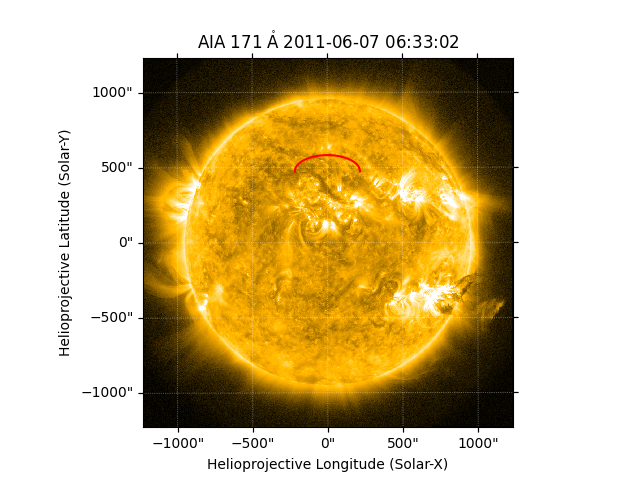In this example we are going to look at saving and loading collections of coordinates with asdf.

asdf is a modern file format designed to meet the needs of the astronomy community. It has deep integration with Python and SunPy and Astropy as well as implementations in other languages. It can be used to store known Python objects in a portable, well defined file format. It is primarily useful for storing complex Astropy and SunPy objects in a way that can be loaded back into the same form as they were saved.

Note

This example requires Astropy 3.1 and asdf 2.3.0

```import numpy as np
import scipy.optimize
import matplotlib.pyplot as plt

import asdf
import astropy.units as u
from astropy.coordinates import SkyCoord

import sunpy.map
from sunpy.sun import constants
from sunpy.coordinates import frames
from sunpy.data.sample import AIA_171_IMAGE
```

Out:

```/home/docs/checkouts/readthedocs.org/user_builds/sunpy/conda/stable/lib/python3.7/site-packages/jsonschema/compat.py:6: DeprecationWarning: Using or importing the ABCs from 'collections' instead of from 'collections.abc' is deprecated, and in 3.8 it will stop working
from collections import MutableMapping, Sequence  # noqa
/home/docs/checkouts/readthedocs.org/user_builds/sunpy/conda/stable/lib/python3.7/site-packages/jsonschema/compat.py:6: DeprecationWarning: Using or importing the ABCs from 'collections' instead of from 'collections.abc' is deprecated, and in 3.8 it will stop working
from collections import MutableMapping, Sequence  # noqa
```

To get started let’s use a function to get the coordinates of a semi-circular loop from this blog post by Will Barnes to generate ourselves some coordinates.

```@u.quantity_input
def semi_circular_loop(length: u.m, latitude: u.deg = 0*u.deg):
"""
Return a Heliographic Stonyhurst coordinate object with points of a semi circular loop in it.
"""

def r_2_func(x):
return np.arccos(0.5 * x / r_sun.to(u.cm).value) - np.pi + length.to(u.cm).value / 2. / x

# Find the loop radius corresponding to the loop length
r_2 = scipy.optimize.bisect(r_2_func,
length.to(u.cm).value / (2 * np.pi),
length.to(u.cm).value / np.pi) * u.cm
alpha = np.arccos(0.5 * (r_2 / r_sun))
phi = np.linspace(-np.pi * u.rad + alpha, np.pi * u.rad - alpha, 2000)

hcc_frame = frames.Heliocentric(
observer=frames.HeliographicStonyhurst(lon=0 * u.deg, lat=latitude, radius=1 * u.AU))

return SkyCoord(
x=r_2 * np.sin(phi),
y=0 * u.cm,
z=r_2 * np.cos(phi) + r_sun,
frame=hcc_frame).transform_to('heliographic_stonyhurst')
```

Use this function to generate a `SkyCoord` object.

```loop_coords = semi_circular_loop(500*u.Mm, 30*u.deg)
print(loop_coords.shape)
# print the first and last coordinate point
print(loop_coords[[0, -1]])
```

Out:

```(2000,)
<SkyCoord (HeliographicStonyhurst: obstime=None): (lon, lat, radius) in (deg, deg, cm)
[(-14.1236018, 29.24437208, 6.957e+10),
( 14.1236018, 29.24437208, 6.957e+10)]>
```

This is a regular coordinate object that can be transformed to other frames or overplotted on images. For instance we could overplot it on an AIA image

```aiamap = sunpy.map.Map(AIA_171_IMAGE)

ax = plt.subplot(projection=aiamap)
aiamap.plot(axes=ax, clip_interval=(1, 99.5) * u.percent)
ax.plot_coord(loop_coords.transform_to(aiamap.coordinate_frame), 'r')

plt.show()
```We can now save these loop points to an asdf file to use later. The advantage of saving them to asdf is that all the metadata about the coordinates will be preserved, and when we load the asdf, we will get back an identical `HeliographicStonyhurst` object.

asdf files save a dictionary to a file, so to save the loop coordinates we need to put them into a dictionary. This becomes what asdf calls a tree.

The asdf file can not save the `SkyCoord` object in versions of Astropy prior to 3.2, but it can save the underlying frame. Therefore we construct a tree with the frame.

```tree = {'loop_points': loop_coords.frame}

with asdf.AsdfFile(tree) as asdf_file:
asdf_file.write_to("loop_coords.asdf")
```

This asdf file is a portable file and can be safely loaded by anyone with Astropy and SunPy installed. We can reload the file like so:

```with asdf.open("loop_coords.asdf") as input_asdf:
coords = input_asdf['loop_points']
new_coords = SkyCoord(coords)

print(new_coords.shape)
# print the first and last coordinate point
print(new_coords[[0, -1]])
```

Out:

```(2000,)
<SkyCoord (HeliographicStonyhurst: obstime=None): (lon, lat, radius) in (deg, deg, cm)
[(-14.1236018, 29.24437208, 6.957e+10),
( 14.1236018, 29.24437208, 6.957e+10)]>
```

Total running time of the script: ( 0 minutes 1.951 seconds)

Gallery generated by Sphinx-Gallery